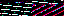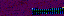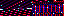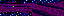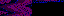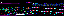```EVR.Text = function(container, text, font, color, size, size_relative)
{
EVR.Component.call(this, container, "p");
!!container.container && (this.size_relative = container.container);
this.set(text, font, color, size, size_relative);
this.style();
this.set_font_size();
this.append();
}
EVR.Text.prototype = new EVR.Component;
EVR.Text.prototype.set = function(text, font, color, size, size_relative)
{
this.element.innerHTML = text;
!!font && (this.font = font);
!!color && (this.color = color);
!!size && (this.size = size);
!!size_relative && (this.size_relative = size_relative);
}
EVR.Text.prototype.style = function()
{
this.css.fontFamily = this.font;
this.css.color = this.color;
this.css.margin = "0px";
}
EVR.Text.prototype.set_font_size = function()
{
var dimensions = this.size_relative.get_dimensions();
var modifier = dimensions / dimensions;
var size = parseInt(dimensions * modifier * this.size);
this.css.fontSize = size + "px";
}
EVR.Text.prototype.get_text = function()
{
return this.element.innerHTML;
}
EVR.Text.prototype.get_font_size = function()
{
return this.css.fontSize.match(/^([0-9]+)px\$/);
}
EVR.Text.prototype.set_line_height = function(height)
{
this.css.lineHeight = parseInt(height) + "px";
}
EVR.Text.prototype.toString = function()
{
return "[object EVR.Text]";
}
```
```EVR.Graphic = function(container, ratio_type, range, alignment, size_relative)
{
EVR.Component.call(this, container, "div");
this.ratio_type = ratio_type || RATIO_INDEPENDENT;
this.range = range || container;
this.size_relative = size_relative || container;
this.aligner = new EVR.Graphic.Aligner(this, alignment);
this.css.position = "absolute";
this.css.fontSize = "0px";
this.offset = [0, 0];
this.proportions = [0, 0];
}
EVR.Graphic.prototype = new EVR.Component;
EVR.Graphic.prototype.set_container = function(container, range)
{
this.container = container;
this.range = range || container;
}
EVR.Graphic.prototype.set_proportions = function(width, height)
{
if (width != null)
{
this.proportions = width;
}
if (height != null)
{
this.proportions = height;
}
}
EVR.Graphic.prototype.get_dimensions = function(ratio)
{
var dimensions = EVR.Component.prototype.get_dimensions.call(this);
if (ratio == true)
{
var relative_dimensions = this.size_relative.get_dimensions();
dimensions /= relative_dimensions;
dimensions /= relative_dimensions;
}
return dimensions;
}
EVR.Graphic.prototype.get_opacity = function()
{
return parseFloat(this.element.style.opacity);
}
EVR.Graphic.prototype.set_text = function(text, font, color, size, relative)
{
relative = relative || this.size_relative;
EVR.Component.prototype.set_text.call(
this, text, font, color, size, relative);
}
EVR.Graphic.prototype.shape = function()
{
var dimensions = this.size_relative.get_dimensions();
var width = dimensions * this.proportions;
var height = dimensions * this.proportions;
if (this.ratio_type == RATIO_WIDTH)
{
height = width * this.proportions;
}
else if (this.ratio_type == RATIO_HEIGHT)
{
width = height * this.proportions;
}
this.set_dimensions(width, height);
}
{
if (this.ratio_type == RATIO_WIDTH)
{
this.proportions += modifier;
}
else if (this.ratio_type == RATIO_HEIGHT)
{
this.proportions += modifier;
}
else
{
this.proportions += modifier;
this.proportions += additional_modifier != null ?
}
this.draw();
}
EVR.Graphic.prototype.append = function()
{
EVR.Component.prototype.append.call(this);
this.draw();
}
EVR.Graphic.prototype.draw = function()
{
this.shape();
this.place();
if (this.text instanceof EVR.Text)
{
this.text.set_font_size();
}
}
EVR.Graphic.prototype.place = function()
{
var coordinates = this.aligner.align();
if (arguments.length > 0 && arguments != null)
{
this.offset = arguments;
}
if (arguments.length > 1)
{
this.offset = arguments;
}
coordinates = this.convert_ratio_to_distance(coordinates);
this.set_coordinates(coordinates);
}
EVR.Graphic.prototype.convert_ratio_to_distance = function(coordinates)
{
var dimensions = this.size_relative.get_dimensions();
coordinates += this.offset * dimensions;
coordinates += this.offset * dimensions;
return coordinates;
}
EVR.Graphic.prototype.move = function()
{
this.offset += arguments;
if (arguments.length > 1)
{
this.offset += arguments;
}
this.place();
}
EVR.Graphic.prototype.toString = function() { return "[object Graphic]"; }
```
```EVR.Graphic.Aligner = function(graphic, alignment)
{
this.graphic = graphic;
this.alignment = alignment || ALIGN_TOP_LEFT;
}
EVR.Graphic.Aligner.prototype.align = function()
{
var coordinates = [0, 0];
//    if (this.alignment == ALIGN_TOP_LEFT)
//    {
//       return coordinates;
//    }
var range = this.graphic.range;
if (range != this.graphic.container)
{
coordinates = range.get_coordinates();
}
switch (this.alignment)
{
case ALIGN_TOP:
coordinates += this.center_x();
break;
case ALIGN_TOP_RIGHT:
coordinates += this.right();
break;
case ALIGN_LEFT:
coordinates += this.center_y();
break;
case ALIGN_CENTER:
coordinates += this.center_x();
coordinates += this.center_y();
break;
case ALIGN_RIGHT:
coordinates += this.right();
coordinates += this.center_y();
break;
case ALIGN_BOTTOM_LEFT:
coordinates += this.bottom();
break;
case ALIGN_BOTTOM:
coordinates += this.center_x();
coordinates += this.bottom();
break;
case ALIGN_BOTTOM_RIGHT:
coordinates += this.right();
coordinates += this.bottom();
break;
}
return coordinates;
}
EVR.Graphic.Aligner.prototype.center_x = function()
{
var range = this.graphic.range;
return range.get_dimensions()/2 - this.graphic.get_dimensions()/2;
}
EVR.Graphic.Aligner.prototype.center_y = function()
{
var range = this.graphic.range;
return range.get_dimensions()/2 - this.graphic.get_dimensions()/2;
}
EVR.Graphic.Aligner.prototype.right = function()
{
var range = this.graphic.range;
return range.get_dimensions() - this.graphic.get_dimensions();
}
EVR.Graphic.Aligner.prototype.bottom = function()
{
var range = this.graphic.range;
return range.get_dimensions() - this.graphic.get_dimensions();
}
EVR.Graphic.Aligner.prototype.toString = function()
{
return "[object EVR.Graphic.Aligner]";
}
```
3.238.70.175
3.238.70.175
3.238.70.175

September 13, 2013

```from array import array
from time import sleep

import pygame
from pygame.mixer import Sound, get_init, pre_init

class Note(Sound):

def __init__(self, frequency, volume=.1):
self.frequency = frequency
Sound.__init__(self, self.build_samples())
self.set_volume(volume)

def build_samples(self):
period = int(round(get_init() / self.frequency))
samples = array("h",  * period)
amplitude = 2 ** (abs(get_init()) - 1) - 1
for time in xrange(period):
if time < period / 2:
samples[time] = amplitude
else:
samples[time] = -amplitude
return samples

if __name__ == "__main__":
pre_init(44100, -16, 1, 1024)
pygame.init()
Note(440).play(-1)
sleep(5)
```

This program generates and plays a 440 Hz tone for 5 seconds. It can be extended to generate the spectrum of notes with a frequency table or the frequency formula. Because the rewards in Send are idealized ocean waves, they can also be represented as tones. Each level has a tone in its goal and a tone based on where the player's disc lands. Both play at the end of a level, sounding harmonic for a close shot and discordant for a near miss. The game can dynamically create these tones using the program as a basis.

I'm also building an algorithmically generated song: Silk Routes (Scissored). Here is an example of how it sounds so far: